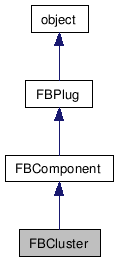# FBCluster Class Reference

FBCluster FBCluster FBCluster FBCluster FBCluster FBCluster FBCluster FBCluster FBCluster FBCluster FBCluster FBCluster FBCluster FBCluster FBCluster FBCluster FBCluster FBCluster FBCluster FBCluster FBCluster FBCluster FBCluster FBCluster FBCluster FBCluster FBCluster FBCluster FBCluster FBCluster FBCluster FBCluster FBCluster FBCluster FBCluster FBCluster FBCluster FBCluster FBCluster FBCluster FBCluster FBCluster FBCluster FBCluster FBCluster FBCluster FBCluster FBCluster FBCluster FBCluster FBCluster FBCluster FBCluster FBCluster FBCluster FBCluster FBCluster FBCluster FBCluster FBCluster FBCluster FBCluster FBCluster FBCluster FBCluster FBCluster FBCluster FBCluster FBCluster FBCluster FBCluster FBCluster FBCluster FBCluster FBCluster FBCluster FBCluster FBCluster FBCluster FBCluster FBCluster FBCluster FBCluster FBCluster FBCluster FBCluster FBCluster FBCluster FBCluster FBCluster FBCluster FBCluster FBCluster FBCluster FBCluster FBCluster FBCluster FBCluster FBCluster FBCluster FBCluster FBCluster FBCluster FBCluster FBCluster FBCluster FBCluster FBCluster FBCluster FBCluster FBCluster FBCluster FBCluster FBCluster FBCluster FBCluster FBCluster FBCluster FBCluster FBCluster
Inheritance diagram for FBCluster:[legend]

List of all members.

## Detailed Description

Cluster.

Weighting interface for meshes. This class is experimental.

## Public Member Functions

int  ClusterBegin (int pIndex=-1)
Begin cluster definition.
int  ClusterEnd (bool pQueryOnly=False)
End cluster definition.
Get the name of a link.
Set the name of a link.
Add a vertex to a cluster.
VertexClear ()
int  VertexGetCount ()
Get the number of vertices.
int  VertexGetNumber (int pIndex)
Get vertex number.
VertexGetTransform (FBVector3d pPosition, FBVector3d pRotation, FBVector3d pScaling)
Get transform of a cluster set.
float  VertexGetWeight (int pIndex)
Get vertex weight.
VertexRemove (int pVertexIndex)
Remove a vertex from a cluster.
VertexSetTransform (FBVector3d pPosition, FBVector3d pRotation, FBVector3d pScaling)
Set transform of a cluster set.
VertexSetWeight (float pWeight, int pIndex)
Set vertex weight.

## Public Attributes

float  ClusterAccuracy
FBClusterMode  ClusterMode

## Member Function Documentation

 int ClusterBegin ( int pIndex = `-1` )

Begin cluster definition.

Parameters:
Returns:
Index of last item(default=-1).

 int ClusterEnd ( bool pQueryOnly = `False` )

End cluster definition.

Parameters:
 pQueryOnly Were the operations on the cluster non-destructive?
Returns:
0, (Not implemented).

 LinkClearUnused ( float pThreshold = `-1.0` )

Parameters:
 pThreshold Weight value under which links are considered unused (default=-1).

Parameters:
Returns:

Returns:

Parameters:
Returns:

Get the name of a link.

Parameters:
Returns:

 int LinkGetVertexIndex ( int pIndex )

Parameters:
 pIndex Index of link to get vertex from.
Returns:
Index value of the current vertex associated to link at index number pIndex

Parameters:

Parameters:

Parameters:
 pModel Model to set.

Set the name of a link.

Parameters:

 VertexAdd ( int pVertexIndex, float pWeight )

Add a vertex to a cluster.

Parameters:
 pVertexIndex Index of vertex to add. pWeight Weight to give to vertex.

 VertexClear ( )

 int VertexGetCount ( )

Get the number of vertices.

Returns:
Number of vertices in a cluster.

 int VertexGetNumber ( int pIndex )

Get vertex number.

Parameters:
 pIndex Index of link to get vertex from.
Returns:
Number value of vertex at link number pIndex

 VertexGetTransform ( FBVector3d pPosition, FBVector3d pRotation, FBVector3d pScaling )

Get transform of a cluster set.

Parameters:
 pPosition Position transform. pRotation Rotation transform. pScaling Scaling transform.

 float VertexGetWeight ( int pIndex )

Get vertex weight.

Parameters:
 pIndex Index of link to get vertex from.
Returns:
Weight of vertex found at link number pIndex.

 VertexRemove ( int pVertexIndex )

Remove a vertex from a cluster.

Parameters:
 pVertexIndex Index of vertex to remove.

 VertexSetTransform ( FBVector3d pPosition, FBVector3d pRotation, FBVector3d pScaling )

Set transform of a cluster set.

Parameters:
 pPosition Position transform. pRotation Rotation transform. pScaling Scaling transform.

 VertexSetWeight ( float pWeight, int pIndex )

Set vertex weight.

Parameters:
 pWeight Weight to give to vertex. pIndex Index of link to get vertex from.

## Member Data Documentation

Generated on Tue Feb 9 19:34:14 2010 for Python Reference Guide by1.5.3